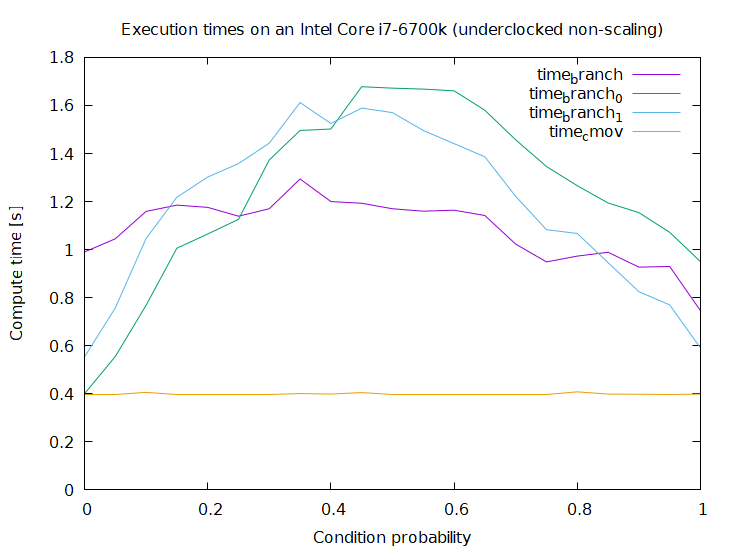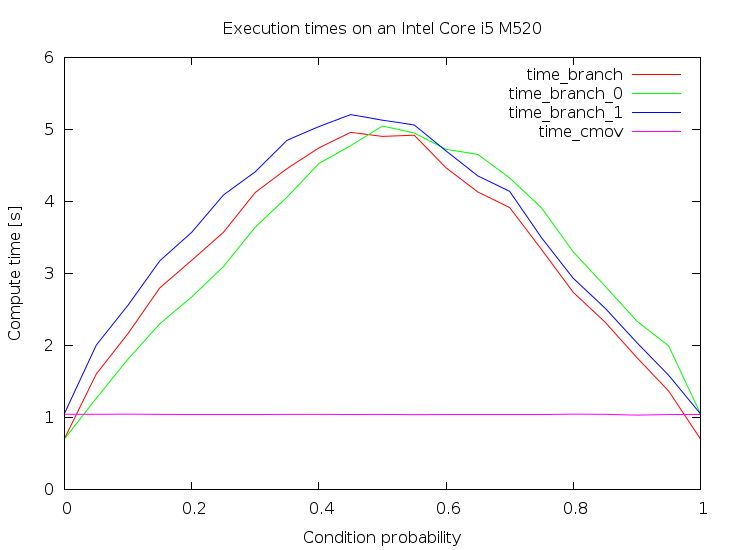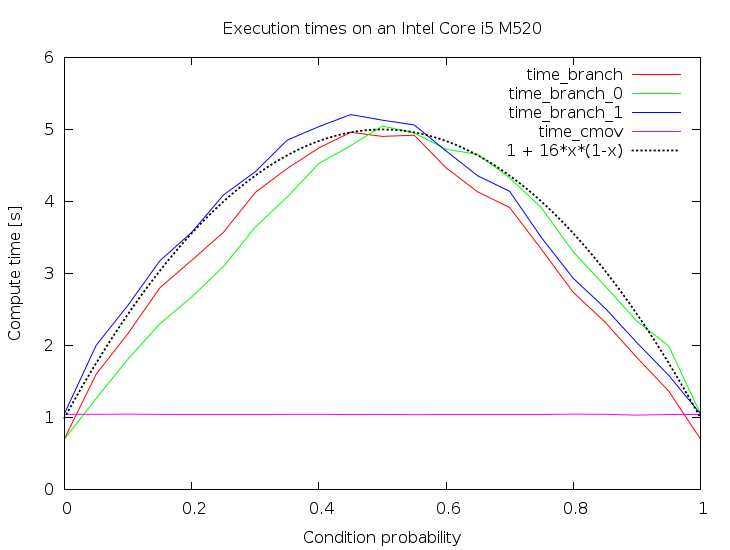/ cmov Public

Measuring cmov vs branch-mov performance

# marcin-osowski/cmov

Switch branches/tags
Nothing to show

A tag already exists with the provided branch name. Many Git commands accept both tag and branch names, so creating this branch may cause unexpected behavior. Are you sure you want to create this branch?

## Files

Failed to load latest commit information.
Type
Name
Commit time

## Comparing the performance of Intel's cmov instruction with a conditional branch + mov pair.

Suppose you want to write the following code inside a performance critical loop:

```    if(cond) {
foo = bar;
}```

where `foo` is a local variable (of some simple type, like `int`), and `bar` is either a local variable, or a simple memory location (such as `A[i]`). Such a code is likely to be found for example in a Kadane's algorithm implementation.

There are two standard ways for an x86-64 compiler to translate this conditional into Intel assembly, namely a `cmov` instruction, or a conditional branch paired with a regular `mov`.

To make this example more concrete I will focus on this particular code:

```    int j;
int res = 0;
for(j = 0; j < n; ++j) {
if(A[j]) {
res = j;
}
}```

GCC version 4.8.2 emits the following code for the `if` at -O3 (gas' assembly notation):

```    movl    (%rdi,%rcx,4), %r9d
testl   %r9d, %r9d
cmovne  %ecx, %eax```

Clearly the compiler stores the location of `A` in `%rdi`, the value of `j` in `%rcx` (`%ecx`), and `res` in `%rax` (`%eax`).

We may however ask it to forget about the `cmov` instruction by using the following command line parameters in addition to -O3: -fno-tree-loop-if-convert -fno-tree-loop-if-convert-stores -fno-if-conversion -fno-if-conversion2. Then we will obtain the following assembly:

```    movl    (%rdi,%rcx,4), %r9d
testl   %r9d, %r9d
je  .L4
movl    %ecx, %eax
.L4:```

To make this example more interesting let's feed the compiler with more data. GCC (and others probably too) implements a mechanism allowing the programmer to specify that a condition will likely/unlikely be true during the runtime. In our case it would look like this (assuming that we are expecting `A[j]` to be generally false):

```    int j;
int res = 0;
for(j = 0; j < n; ++j) {
if(__builtin_expect(A[j], 0)) {
res = j;
}
}```

The corresponding GCC output:

```    movl    (%rdi,%rcx,4), %r9d
testl   %r9d, %r9d
jne .L20
.L14:
/* Other code */
.L20:
movl    %ecx, %eax
jmp .L14```

We are of course free to put `1` in the place of `0` whenever we expect `A[j]` to be true most of the time:

```    int j;
int res = 0;
for(j = 0; j < n; ++j) {
if(__builtin_expect(A[j], 1)) {
res = j;
}
}```

And the corresponding GCC output (note the speculative `movl` execution!):

```    movl    %eax, %r8d
movl    (%rdi,%rcx,4), %r10d
movl    %ecx, %eax
testl   %r10d, %r10d
je  .L29
.L24:
/* Other code */
.L29:
movl    %r8d, %eax
jmp .L24```

This way we obtain four versions of the program which I will call cmov, branch, branch_0 and branch_1. One might ask why there is no cmov_0 and no cmov_1, but it is due to the fact that in cmov's case the compiler ignored the likely/unlikely hints.

The point of this excercise is to measure their execution speed, but this of course depends on the contents of the `A` array. So let's fill it with random independent identically distributed zeros and ones, manipulating the probability of one (the probability that the if's condition will be true).

# Update 2018:

Thanks to AndreasPK for providing this data.

On an i7-6700k (Skylake) cmov is now faster under all circumstances:While the performance at the extremes looks the same cmov is still faster which becomes obvious when looking at the raw numbers:

``````0.000000;0.983000;0.412000;0.550000;0.411000
...
1.000000;0.747000;0.951000;0.591000;0.398000
``````

branch_0 comes (very) close for probability zero but was still always slightly slower than cmov in 10 tests I ran.

# Original Results

These are the results I obtained on my machine. It is the execution time, so the lower the better:Three interesting facts can be read from this plot:

• The `cmov` instruction is generally faster. It is also immune to the probability
• However the branch version is faster for stable, predictable conditions.
• The branch versions take approximately `p*(1-p)` time to execute. The nice parabola-alike looks is approximately the variance of the distribution that `A` was sampled from.

The "visual proof" for the third fact:To conclude, GCC emits `cmov` by default, and it seems like a reasonable choice, at least on my Intel Core i5. However, as noted before, it is not the optimal solution for stable, predictable conditions.

Measuring cmov vs branch-mov performance

## Releases

No releases published

## Packages 0

No packages published

•
•